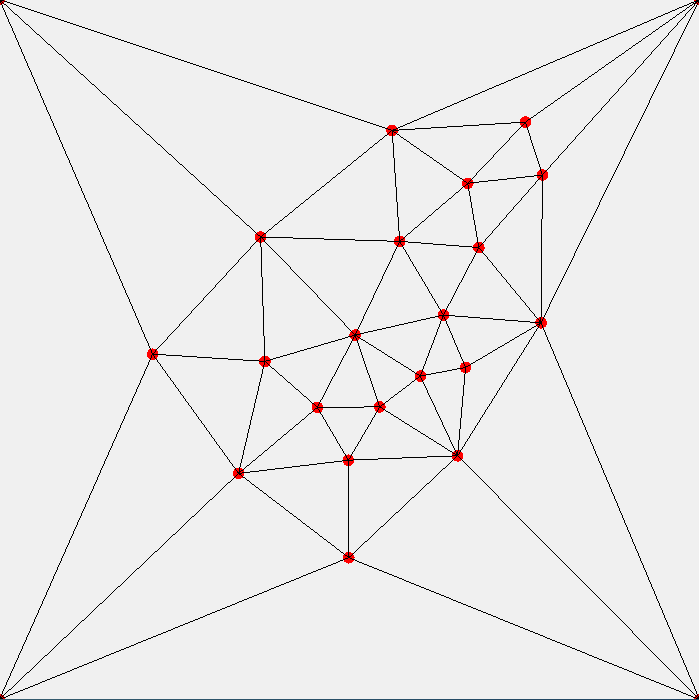Main page Blogs

# Deforming a random Delaunay triangulation into its Tutte embedding

Mathias Fuchs, February 2019
I was trying to set up a suitable notion of Laplacian matrix for Tutte embeddings of graphs.
Suppose, as usual, we have a graph with $V$ vertices, with $n_v$ being the degree of vertex $v$. Then, the condition is that each vertex be the average of its neighbors. So, each vertex contributes three conditions, i.e., three rows to the constraint matrix. Thus, we end up with a matrix whose number of columns is $3V$, three for each vertex, and likewise for the number of rows. The three rows of our Laplacian contributed by a vertex without positional constraint look as follows: $$L = \left ( \begin{matrix} \cdots\\ \cdots\\ -1 & 0 & 0 & \cdots & n_v & 0 & 0 & \cdots & -1 & 0 & 0 & \cdots\\ 0 & -1 & 0 & \cdots & 0 & n_v & 0 & \cdots & 0 & -1 & 0 & \cdots\\ 0 & 0 & -1 & \cdots & 0 & 0 & n_v & \cdots & 0 & 0 & 1 & \cdots\\ \cdots \\ \cdots \\ \end{matrix} \right )$$ In each row, the sum is zero because the $-1$'s add up to $-n_v$. Thus, we are dealing with a singly-stochastic matrix.
However, we need to take care of vertices whose positions are fixed. Such a vertice's three-row block of $L$ looks as follows instead: $$L = \left ( \begin{matrix} \cdots\\ \cdots\\ 0 & 0 & 0 & \cdots & 1 & 0 & 0 & \cdots\\ 0 & 0 & 0 & \cdots & 0 & 1 & 0 & \cdots\\ 0 & 0 & 0 & \cdots & 0 & 0 & 1 & \cdots\\ \cdots \\ \cdots \\ \end{matrix} \right)$$ and the corresponding three entries of the right-hand side $a$ contain the three fixed coordinates, making the linear system $$Lx = a$$ inhomogeneous.
And there we are!Figure of a mesh whose vertices are solutions to $Lx = a$, with $a$ dictated by the square corners, and the topology of the mesh given by the Delaunay triangulation of a random set of vertices.